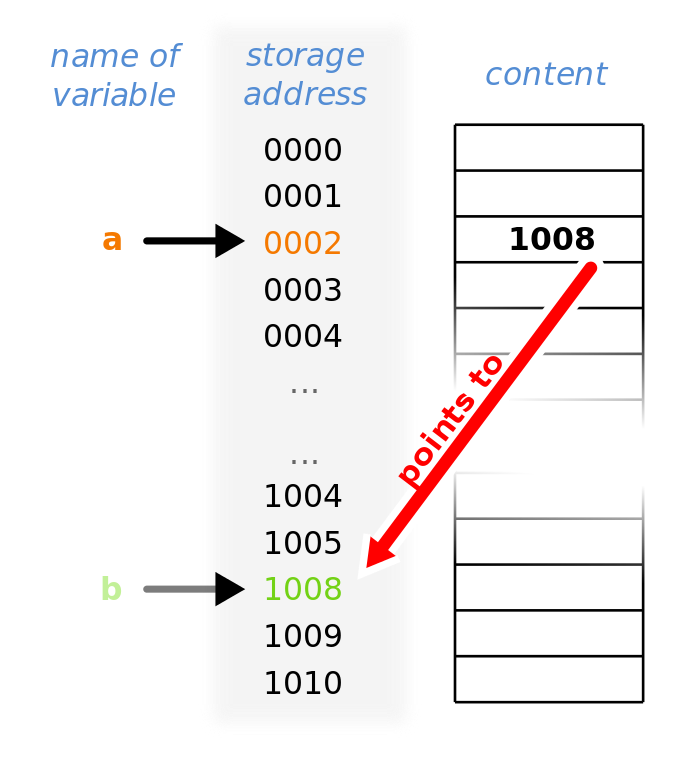# Pointers¶

Cyganek section 3.12

Pointers are similar to references in that they provide indirect access to an object’s data. However, in C++, references are much more widely used than pointers.

Note

References and pointers can provide similar functionality. In general, pointers are more general but also more error-prone. Here’s a summary of the differences between references and pointers

Here’s a simple example:

int *a;
int b;

a = &b;


This has the pointer a point to the memory location of b (we use the address operator & here). Visually, this appears as:Fig. 8 A pointer, a that points to the memory location of variable b.¶ (Wikipedia/Sven)

We can access the data pointed to by the pointer by using the dereference operator, *. Here’s an example:

Listing 16 pointers_example.cpp
#include <iostream>

int main() {

double x{2.0};

double *x_ptr;

double &x_ref = x;

std::cout << "x = " << x << std::endl;

// we can access x through the reference x_ref

x_ref *= 2.0;

std::cout << "x = " << x << std::endl;
std::cout << "x_ref = " << x_ref << std::endl;

// now let's associate x_ptr with x

x_ptr = &x;

// to access the data pointed to by x_ptr, we need to
// dereference it

std::cout << "x_ptr = " << x_ptr << std::endl;
std::cout << "*x_ptr = " << *x_ptr << std::endl;

// likewise, to modify its value

*x_ptr *= 2;

std::cout << "x = " << x << std::endl;
std::cout << "x_ref = " << x_ref << std::endl;

}


Note

We will not use pointers directly much in this class, but they are useful for managing memory. Later (if there is time) we’ll see that there are smart pointers in C++ that handle a lot of the memory management for us.

Tip

It is a good idea to initialize all pointers (just like we do for all other object types). C++ provides nullptr for this reason:

int *p = nullptr;


This then allows us to use the pointer in a conditional, like:

if (p) {
// do stuff
}


try it…

What happens if we compare two pointers with ==?